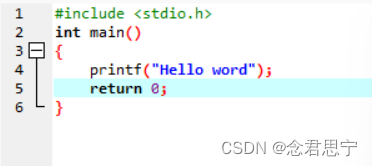+关注继续查看

# 第1章 C语言概述

1.1和1.2自己看吧........
1.3 简单的C语言程序介绍

# 例1.1

#include<stdio.h>
void main() {

printf("This is a C program!/n");

}

1。关于#include<stdio.h>
void main(){.........}

让我们从向黑板上书写文字开始吧.......
我们写信有一定的格式， 大致分为3部分：开头、正文和结尾。
信是我们写来给别人看得，大家都使用诸如上面的格式， 无论什么人拿到信，均能很快知道， 是谁写的信，写给谁的，主要说的什么。 由此可见，人和人之间沟通的书面文字是有一定书写规范的。
那么同样的道理，作为人类和计算机之间沟通的书面文字(实质是就是 人向机器发出的命令的集合体——程序)， 也要遵循一定的格式，而且 简单的C程序也大致分为3部分：开头、正文和结尾，和信比较如下图 ：

XXX，你好：        -----------开头-----------    #include<stdio.h>
void main(){

。。。              -----------正文------------    。 。 。

此致

XXX
XX年XX月XX日

(1) 开头: #include<stdio.h>
void main(){                                相当于    XXX，你好：

(2)正文: 你要让机器执行的命令写在开头和结尾之间      相当于    信的正文内容部分

(3)结尾: }                                        相当于    此致 敬礼 XXX XX年XX月XX日

现在要在黑板上写个星号*          现在要让在屏幕上写个星号*

对方:人 , 表达形式:信                对方:计算机 , 表达形式:c语言

xxx 你好:                                     #include<stdio.h>
void main(){

在黑板上写一个*号                    printf("*");

此致                                          }
敬礼
xxx
XX年XX月XX日

就像人类信的开头和结尾部分一般都是固定的一样,c语言程序的开始和结束部分一般也是固定的,简单程序更是如此,
开头照抄 #include void main(){    , 结尾照抄一个大括号 } .

比如 现在我要让在屏幕上写个#的程序如下 用c语言

#include<stdio.h>      ------------开头两句照抄
void main(){
printf("#"); ------------屏幕书写#的命令
}             -----------结尾用个右大括号

再比如 现在我要让在屏幕上写两个井号#的程序如下 用c语言

#include<stdio.h> ------------开头两句照抄
void main(){

printf("##");     ------------屏幕书写#的命令
}         ----------- 结尾用个右大括号

我们看到程序是如此的相似, 开头和结尾完全相同,

# 2.关于printf

屏幕书写命令是printf("XXXX");其中XXXX就是向屏幕上书写的内容
比如 在屏幕上写两个星 ** 的程序如下 ,c语言

#include<stdio.h> ------------开头两句照抄
void main(){
printf("**");     ------------屏幕书写**的命令
}                 ----------- 结尾用个右大括号

我们让计算机向屏幕上书写的术语叫 "输出",而且可以向屏幕输出的内容 可以是字母,数字以及#!@\$%&^&*)(*_等等好多东西.
现在我们让计算机向屏幕上输出一串英文文字"This is a C program !" , C程序显然如下:

#include<stdio.h> ------------------开头两句照抄
void main(){
printf("This is a C program !");---屏幕输出命令
}                       -----------结尾用个右大括号# 3. 关于'/n'

*
* *
* * *

#include<stdio.h>
void main(){
printf("*");
printf("* *");
printf("* * *");
}为什么会这样呢？解释这个问题必须先说明一些关于屏幕的基础知识。。。。。

早期计算机的屏幕整个区域被分成2580列，如下所示：总共80x25个位置，每个位置可以放一个字符。

设列为x轴,行为y轴,原点是屏幕左上角，用(x,y)表示屏幕上第x列第y行的输出位置，比如第3列，第5行表示为（3，5），注意：（列，行）

假设系统最初将当前输出位置设定在（1，1），printf将在以（1，1），（2，1），（3，1）（4，1）.....的顺序从左到右放置输出内容，直到第一行最右端的（80，1），再从第2行最左端的（1，2）开始显示，（2，2），（3，2），.......,当第2行放满，再放第3行,......如此直到25行全部写满后，上卷屏。#include<stdio.h>
void main( ){

printf("_ _ * _ _ _ _ _ _ _ _ _ ......_ _ _ _ _ _ _");
/*星号后面有80-3个空格*/

printf("_ * _ * _ _ _ _ _ _ _ _ ......_ _ _ _ _ _ _");
/*第2个星号后面有80-4个空格*/

printf("* _ * _ * _ _ _ _ _ _ _ ......_ _ _ _ _ _ _");
/*第3个星号后面有80-5个空格*/

}

#include<stdio.h>
void main(){

printf("_ _ * /n");
/*在从(1,1)开始先输出_ _ *，然后'/n'将当前输出位置移动到第2行的行首(1,2)*/

printf("_ *_ * /n");
/*在从(1,2)开始先输出 * _ *_ *，然后'/n'将当前输出位置移动到第3行的行首(1,3)*/

printf("* _ *_ * /n");
/*在从(1,3)开始先输出 * _ * _ *，然后'/n'将当前输出位置移动到第3行的行首(1,4)*/

}

#include<stdio.h>
void main(){

gotoxy(5,5);
printf("*");

}

1。关于简单C程序的基本结构的介绍
#include<stdio.h>
void main(){.........}

2。关于屏幕输出的基础知识和printf

3. 关于 '/n'的应用

#include<stdio.h>
void main(){
int a,b,sum;
a=123;
b=456;
sum=a+b;
printf("sum=%d/n",sum);
}

1.设有3个变量a,b,sum，（a,b,sum均∈整数）
2.已知 a=123,b=456, 有 sum=a+b
3.求 sum
4.答案不要告诉我，而是把结果写在黑板上，

对方：人                                                              对方：计算机

设有3个变量a,b,sum,（a,b,sum均∈整数）        int a,b,sum;/*int 表示后面写的3个是整数变量*/

已知 a=123,b=456,sum=a+b 求 sum                  a=123,b=456;sum=a+b;

答案不要告诉我，而是把结果写在黑板上           printf("sum=%d",sum);

写时用"sum=?"的格式, ?用sum具体值代替                   /*printf表示向屏幕写，

写时用"sum=%d"的格式,

%d用sum具体值代替,%d就是？*/

2.任意给定 a和 b, 有sum=a+b

1. 第1句，
乙了解到将有3个变量，这3个变量将用于整数计算。

2. 第2句，
乙了解到此时需要让甲给出a和b的具体整数值，因此乙将一直等待，当甲给出a和b的具体的整数值后，乙继续进行解题，即进入第3步。

3. 第3句，
乙将a和b相加求出sum的值

4. 第4句，

a=123;b=456;          改成          scanf("%d%d",&a,&b);

#include<stdio.h>
void main(){
int a,b,sum;
scanf("%d%d",&a,&b);
sum=a+b;
printf("sum=%d/n",sum);
}

123 456 ↓      /*↓表示按Enter键，即按回车键*/

sum=579#include<stdio.h >
void main(){
int max(int x,int y);
int a,b,c;
scanf("%d,%d",&a,&b);
c=max(a,b);
printf("max=%d/n",c);
}

int max(int x, int y)
{
int z;
if(x>y)z=x;
else z=y;
return z;
}<;p>      *
* *
* * *

#include<stdio.h>
void main(){

printf("* * * * * * * * * * * * * * * * * * * * * * * * * * * * * * /n");

printf("                        Very good!/n");

printf("* * * * * * * * * * * * * * * * * * * * * * * * * * * * * * /n");

}

1.给你一个标准c的较经典并且好理解的解答：
int max(int x,int y)
{
int z;
if(x>y)z=x;
else z=y;
return z;
}
main()
{
int a,b,c,max_abc;
scanf("%d%d%d",&a,&b,&c);
max_abc=max(a,b);
max_abc=max(max_abc,c);
printf("max=%d",max_abc);
}

2.求最大值，用函数的较精简的写法
int max(int x,int y)
{
int z;
if(x>y)z=x;
else z=y;
return z;
}

main()
{
int a,b,c,maxium;
scanf("%d%d%d",&a,&b,&c);
maxium=max(max(a,b),c); /*a和b大的那个数和c比较大小*/
printf("%d",maxium);/*输出a,b,c三个当中最大的那个数*/
getch();
}

3 要说写法较简单的,给你一个
main()
{
int a,b,c,max;
scanf("%d%d%d",&a,&b,&c);
printf(" max=%d",((max=(a>b)?a:b))>c?max:c);
getch();
}

4 实质相同的好理解的,给你一个
main()
{
int a,b,c,max;
scanf("%d,%d,%d",&a,&b,&c);

if(a<b) max=b;
else    max=a;

if(max<c)
max=c;

printf("max=%d/n",max);
}

5 要输出最大值和最小值怎么写?

#include<stdio.h>
int max(int x,int y)
{
int z;
if(x>y)z=x;
else z=y;
return z;
}
int min(int x,int y)
{
int z;
if(x<y)z=x;
else z=y;
return z;
}
main()
{
int a,b,c,max_abc,min_abc;
scanf("%d%d%d",&a,&b,&c);
max_abc=max(a,b);
max_abc=max(max_abc,c);
printf("max=%d ",max_abc);
min_abc=min(a,b);
min_abc=min(min_abc,c);
printf(" min=%d/n",min_abc);
getch();
}

6 要输出a,b,c,d,e,f,g中的最大值和最小值怎么写?
int max(int x,int y)
{
int z;
if(x>y)z=x;
else z=y;
return z;
}
int min(int x,int y)
{
int z;
if(x<y)z=x;
else z=y;
return z;
}
main()
{
int a,b,c,d,e,f,g,max_abc,min_abc;
scanf("%d%d%d%d%d%d%d",&a,&b,&c,&d,&e,&f,&g);
max_abc=max(a,b);
max_abc=max(max_abc,c);
max_abc=max(max_abc,d);
max_abc=max(max_abc,e);
max_abc=max(max_abc,f);
max_abc=max(max_abc,g);
printf("max=%d ",max_abc);
min_abc=min(a,b);
min_abc=min(min_abc,c);
min_abc=min(min_abc,d);
min_abc=min(min_abc,e);
min_abc=min(min_abc,f);
min_abc=min(min_abc,g);
printf(" min=%d/n",min_abc);
}

7.输入a,b,c,输出最大值MAX,中间值MID,最小值MIN
#include <stdio.h>
main()
{
int a,b,c;
int t;
printf("/nintput a :");
scanf("%d",&a);
printf("/nintput b :");
scanf("%d",&b);
printf("/nintput c :");
scanf("%d",&c);
/*将a,b,c的值按由大到小重新排列*/
if(a<b){t=a;a=b;b=t;}
if(a<c){t=a;a=c;c=t;}
if(b<c){t=b;b=c;c=t;}
printf("max: %d mid: %d min: %d /n",a,b,c);
getch();
}

8 请问有更经典的解法吗？ 学习了循环和数组以后，再。。。。。。。。。

OVER1896 01164 0《C语言编程——零基础初学者指南(第3版)》一第2章 编写第一个C程序2.1　概述
1784 0C语言及算法设计课程实验三：最简单的C程序设计——顺序程序设计(四)
C语言及算法设计课程实验三：最简单的C程序设计——顺序程序设计(四)
40 0C语言及算法设计课程实验三：最简单的C程序设计——顺序程序设计(三)
C语言及算法设计课程实验三：最简单的C程序设计——顺序程序设计(三)
31 0C语言及算法设计课程实验三：最简单的C程序设计——顺序程序设计(二)
C语言及算法设计课程实验三：最简单的C程序设计——顺序程序设计(二)
66 0C语言程序设计教程任务；初步认识C语言，携君之手，步入编程之路。
C语言程序设计教程任务；初步认识C语言，携君之手，步入编程之路。
40 0
rdiframework
RDIFramework.NET，基于.NET的快速信息化系统开发、整合框架，给用户和开发者最佳的.Net框架部署方案。 框架官网：http://www.rdiframework.net/ 框架博客：http://blog.rdiframework.net/

C语言学习教程189641281534044

Python语言基础 - 函数、面向对象、异常处理5474753

Python语言基础 - 语法入门9077657

Go语言核心编程 - 数据结构和算法159747

Go语言核心编程 - 面向对象、文件、单元测试、反射、TCP编程1316162